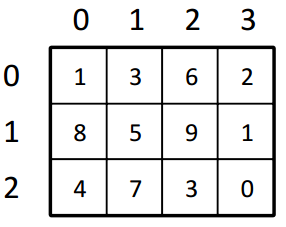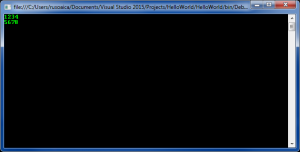Sunday, September 24, 2023 21:45

## Multidimensional arrays

In mathematics, one-dimensional arrays are also known as vectors. Some of you may still remember (riiight! 🙂 ) that multidimensional arrays are called matrices. In programming, we call multidimensional arrays any array with more than one dimension.

For instance, how would we represent the structure of a chess board programmatically? It has two dimensions: one of numbers (starting from one, ending with 8) and one of letters (from A to H), and we identify a cell by the two combined, like in a system of coordinates: H3, B6, etc.

The way we achieve this programatically is by declaring a two dimensional array, like this:

If you want to represent a three dimensional system, like the coordinates of a 3D point (X, Y and Z axis) you can declare a three-dimensional array:

and so on. However, two dimensional arrays are rarely used, and more than two dimensions ever more rarely. However, in theory, you could declare an infinite number of dimensions.

We declare multidimensional array like so:

and we can initialize them like this:

Because multidimensional arrays are hard to understand as a concept seen like that, so you can better imagine and visualize them by the following image:The above image represents the visual representation of our intMatrix array, populated with some random elements. As you can see from our initialization, we declared intMatrix with two dimensions, one of 3 elements, one of 4. The image illustrates the same exact thing, keeping into account that multidimensional arrays, just like regular arrays, are 0 based indexed.

Lastly, this is how we initialize our multidimensional arrays with some values:

And yes, it is a bit complicated. But don’t feel bad, even experienced programmers have problems conceptualizing more than three dimensional arrays 😀

However, accessing or setting the value of specific locations of multidimensional arrays is a bit more simpler. Lets consider the following declaration:

The array _matrix has a total of 8 elements, 2 rows with 4 columns each. So, its elements can be accessed like this:

After a quick inspection of my example, you will probably figure out that two dimensional arrays can be accessed by

and similarly, any number of dimensions can be accessed with

Another concept you should be aware of is the length of multidimensional arrays. Of course, we cant access a Length property directly, like we used for a one-dimensional array, that is not possible. That is because each dimension has its own length, which can be retrieved this way:

At a first sight, you’d think that _matrix.GetLength(0) would return the length of the first row, while _matrix.GetLength(1); the values of the second, and that would be a nasty trap! Actually, _matrix.GetLength(0) returns the length of our first dimension – the rows – which in our case is 2, while _matrix.GetLength(1) returns the length of our second dimension – the columns – which is 4!

To conclude this lesson, this is how we display the elements of a matrix to the console:

First we declare and initialize an array. The array is two-dimensional, therefore we use a for-loop which will iterate through the rows, and a nested for loop which for each row will iterate through the columns. At each iteration we will print the current element using the appropriate method to access this element by using its two indices (row and column). Finally, if we execute this piece of code we will get the following result:The concepts explained in this lesson are also shown visually as part of the following video: